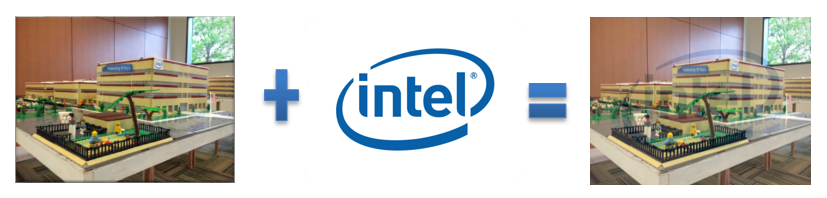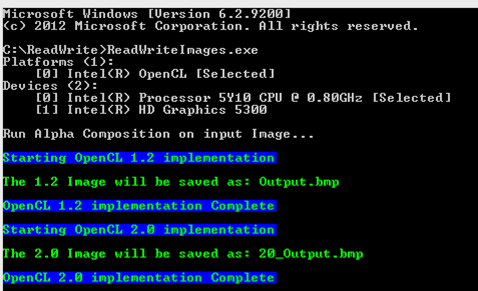# Using OpenCL™ 2.0 Read-Write Images

ID 673129
Updated 7/29/2015
Version Latest
PublicBy

## Acknowledgements

We want to thank Javier Martinez, Kevin Patel, and Tejas Budukh for their help in reviewing this article and the associated sample.

## Introduction

Prior to OpenCL™ 2.0, there was no ability to read and write to an image within the same kernel. Images could always be declared as a “CL_MEM_READ_WRITE”, but once the image was passed to the kernel, it had to be either “__read_only” or “__write_only”.

 input1 = clCreateImage( oclobjects.context, CL_MEM_READ_WRITE|CL_MEM_COPY_HOST_PTR, &format, &desc, &input_data1, &err ); SAMPLE_CHECK_ERRORS( err ); 

Code 1. Image buffer could be created with CL_MEM_READ_WRITE

 __kernel void Alpha( __read_write image2d_t inputImage1, __read_only image2d_t inputImage2, uint width, uint height, float alpha, float beta, int gamma ) 

Code 2. OpenCL 2.0 introduced the ability to read and write to images in Kernels

The addition, while intuitive, comes with a few caveats that are discussed in the next section.

## The value of Read-Write Images

While Image convolution is not as effective with the new Read-Write images functionality, any image processing technique that needs be done in place may benefit from the Read-Write images. One example of a process that could be used effectively is image composition.

In OpenCL 1.2 and earlier, images were qualified with the “__read_only” and __write_only” qualifiers. In the OpenCL 2.0, images can be qualified with a “__read_write” qualifier, and copy the output to the input buffer. This reduces the number of resources that are needed.

Since OpenCL 1.2 images are either read_only or write_image. Performing an in-place modifications of an image requires treating the image as a buffer and operating on the buffer (see cl_khr_image2d_from_buffer: /content/www/us/en/develop/articles/using-image2d-from-buffer-extension.html.

The current solution is to treat the images as buffers, and manipulate the buffers. Treating 2d images as buffers many not be a free operation and prevents clamping and filtering abilities available in read_images from being used. As a result, it may be more desirable to use read_write qualified images.

## Overview of the Sample

The sample takes two windows bitmap images “input1.bmp” and “input2.bmp” and puts them into an image buffer. These images are then composited based on the value of the alpha, a weight factor in the equation of the calculated pixel, which can be passed in as an option.Figure 1. Using Alpha value 0.84089642

The images have to be either 24/32-bit images. The output is a 24-bit image. The images have to be of the same size. The images were also of the Format ARGB, so when loading that fact was taken into consideration.Figure 2. Using Alpha value of 0.32453

The ARGB is converted to RGBA. Changing the value of the beta value causes a significant change in the output.

## Using the Sample SDK

The SDK demonstrates how to use image composition with Read write images. Use the following command-line options to control this sample:

 Options Description -h, --help Show this text and exit -p, --platform number-or-string Select platform, devices of which are used -t, --type all | cpu | gpu | acc | default | Select the device by type on which the OpenCL Kernel is executed -d, --device number-or-string Select the device on which all stuff is executed -i, --infile 24/32-bit .bmp file Base name of the first .bmp file to read. Default is input1.bmp -j, --infile 24/32-bit .bmp file Base name of the second .bmp file to read Default is input2.bmp -o, --outfile 24/32-bit .bmp file Base name of the output to write to. Default is output.bmp for OCL1.2 and 20_output.bmp for OCL2.0 -a, --alpha floating point value between 0 and 1 Non-zero positive value that determines how much the two images will blend in composition. Default alpha is 0.84089642. Default beta value is 0.15950358.

The sample SDK has a number of default values that allow the application to be able to run without any user input. The user will be able to use their input .bmp files. The files have to be either 24/32 bmp files as well. The alpha value is used to determine how much prominence image one will have over image 2 as such:

calculatedPixel = ((currentPixelImage1 * alpha) + (currentPixeImage2 * beta) + gamma);

The beta value is determined by subtracting the value of the alpha from 1.

float beta = 1 – alpha;

These two values determine the weighted distribution of images 1 to image 2.

The gamma value can be used to brighten each of the pixels. The default value is 0. But user can brighten the overall composited image.

## Example Run of ProgramFigure 3. Program running on OpenCL 2.0 Device

Barriers cannot be used with images that require synchronization across different workgroups. Image convolution requires synchronizing all threads. Convolution with respect to images usually involves a mathematical operation on two matrices that results in the creation of a third matrix. An example of an image convolution is using Gaussian blur. Other examples are image sharpening, edge detection, and embossing.

Let’s use Gaussian blur as an example. A Gaussian filter is a low pass filter that removes high frequency values. The implication of this is to reduce detail and eventually cause a blurring like effect. Applying a Gaussian blur is the same as convolving the image with a Gaussian function that is often called the mask. To effectively show the functionality of Read-Write images, a horizontal and vertical blurring had to be done.

In OpenCL 1.2, this would have to be done in two passes. One kernel would be exclusively used for the horizontal blur, and another does the vertical blur. The result of one of the blurs would be used as the input of the next one depending on which was done first.

 __kernel void GaussianBlurHorizontalPass( __read_only image2d_t inputImage, __write_only image2d_t outputImage, __constant float* mask, int maskSize) {     int2 currentPosition = (int2)(get_global_id(0), get_global_id(1));     float4 currentPixel = (float4)(0,0,0,0);     float4 calculatedPixel = (float4)(0,0,0,0);     for(int maskIndex = -maskSize; maskIndex < maskSize+1; ++maskIndex)     {         currentPixel = read_imagef(inputImage, imageSampler, currentPosition + (int2)(maskIndex, 0));         calculatedPixel += currentPixel * mask[maskSize + maskIndex];     }     write_imagef(outputImage, currentPosition, calculatedPixel); } __kernel void GaussianBlurVerticalPass( __read_only image2d_t inputImage, __write_only image2d_t outputImage, __constant float* mask, int maskSize) {     int2 currentPosition = (int2)(get_global_id(0), get_global_id(1));     float4 currentPixel = (float4)(0,0,0,0);     float4 calculatedPixel = (float4)(0,0,0,0);      for(int maskIndex = -maskSize; maskIndex < maskSize+1; ++maskIndex)     {         currentPixel = read_imagef(inputImage, imageSampler, currentPosition + (int2)(0, maskIndex));         calculatedPixel += currentPixel * mask[maskSize + maskIndex];     }     write_imagef(outputImage, currentPosition, calculatedPixel); } 

Code 3. Gaussian Blur Kernel in OpenCL 1.2

The idea for the OpenCL 2.0 would be to combine these two kernels into one. Use a barrier to force the completion of each of the horizontal or vertical blurs before the next one begins.

 __kernel void GaussianBlurDualPass( __read_only image2d_t inputImage, __read_write image2d_t tempRW, __write_only image2d_t outputImage, __constant float* mask, int maskSize) {     int2 currentPosition = (int2)(get_global_id(0), get_global_id(1));     float4 currentPixel = (float4)(0,0,0,0);       float4 calculatedPixel = (float4)(0,0,0,0)     currentPixel = read_imagef(inputImage, currentPosition);     for(int maskIndex = -maskSize; maskIndex < maskSize+1; ++maskIndex)     {         currentPixel = read_imagef(inputImage, currentPosition + (int2)(maskIndex, 0));              calculatedPixel += currentPixel * mask[maskSize + maskIndex];     }     write_imagef(tempRW, currentPosition, calculatedPixel);     barrier(CLK_GLOBAL_MEM_FENCE);     for(int maskIndex = -maskSize; maskIndex < maskSize+1; ++maskIndex)     {         currentPixel = read_imagef(tempRW, currentPosition + (int2)(0, maskIndex));         calculatedPixel += currentPixel * mask[maskSize + maskIndex];     }     write_imagef(outputImage, currentPosition, calculatedPixel); } 

Code 4. Gaussian Blur Kernel in OpenCL 2.0

Barriers were found to be ineffective. Using a barrier does not guarantee that the horizontal blur is completed before the vertical blur begins, assuming you did the horizontal blur first. The implication of this was an inconsistent result in multiple runs. Barriers can be used to synchronize threads within a group. The reason the problem occurs is that edge pixels are read from multiple workgroups, and there is no way to synchronize multiple workgroups. The initial assumption that we can implement a single Gaussian blur using read_write images proved incorrect because the inter-workgroup data dependency cannot be synchronized in OpenCL.

## References

Oludemilade Raji is a Graphics Driver Engineer at Intel’s Visual and Parallel Computing Group. He has been working in the OpenCL programming language for 4 years and contributed to the development of the Intel HD Graphics driver including the development of OpenCL 2.0.

Robert Ioffe is a Technical Consulting Engineer at Intel’s Software and Solutions Group. He is an expert in OpenCL programming and OpenCL workload optimization on Intel Iris and Intel Iris Pro Graphics with deep knowledge of Intel Graphics Hardware. He was heavily involved in Khronos standards work, focusing on prototyping the latest features and making sure they can run well on Intel architecture. Most recently he has been working on prototyping Nested Parallelism (enqueue_kernel functions) feature of OpenCL 2.0 and wrote a number of samples that demonstrate Nested Parallelism functionality, including GPU-Quicksort for OpenCL 2.0. He also recorded and released two Optimizing Simple OpenCL Kernels videos and GPU-Quicksort and Sierpinski Carpet in OpenCL 2.0 videos.

You might also be interested in the following:

Optimizing Simple OpenCL Kernels: Modulate Kernel Optimization

Optimizing Simple OpenCL Kernels: Sobel Kernel Optimization

GPU-Quicksort in OpenCL 2.0: Nested Parallelism and Work-Group Scan Functions

Sierpiński Carpet in OpenCL 2.0